Perspective

Author V. C. Mohan
Date Aug 23, 2008

This plugin converts a Perspective iview image to its front view or vice versa. This plugin employs different technique than that of Reform plugin. The image is assumed to be 2D plane.

Coefficients for x (and y) axis, coordinates of camera position need to be specified for correction. While for small coefficients the process of skew<>deskew is reversible by just changing sign of coefficients, for larger coefficients values it is not. A z coordinate for camera can also be specified. If it is non zero then only coefficient a is used for all dimensions.

It is assumed that several vertical and horizontal lineaments are present in the image and are distorted due to position of camera. These need not form a single rectangle. In test mode a grid of perspective view dotted lines are superimposed on image. Along test clip (generated with the single specified frame), The parameters (coefficients, camera position coordinates) are varied linearly and the resulting Perspective view dotted lines are generated. These displays may aid in selecting proper values of parameters.

By using suitable values for z and ez (also if needed x, ex, y and ey) and a, effect of zoom in or zoom out can be produced.

 Description Name Type Limits Default Input clip clip RGB format only none coefficient a (along width or for all) a float between - 0.5 and 0.5 -0.0005 coefficient b(along height only) b float between - 0.5 and 0.5 a camera location x coord relative to frame coordinates) x integer between minus frame width and twice frame width 0 camera location y coord relative to frame coordinates) y integer between minus frame height and twice frame width frame height camera location z coord relative to frame ) z integer zero or any positive number 0 whether in test mode test Boolean true for test, false for normal false end coefficient ea (along width)test mode only ea float between - 0.5 and 0.5 -0.0005 end coefficient eb(along height only )test mode only eb float between - 0.5 and 0.5 0.0005 end camera location x coord relative to frame coordinates)both modes ex integer between minus frame width and twice frame width x end camera location y coord relative to frame coordinates)both modes ey integer between minus frame height and twice frame width y end camera location z coord relative to frame both modes ez integer zero or any positive number z number of steps for variation ( in test mode only) nsteps integer between 0 and 100 20 input frame number for over lay ( in test mode only) frame Int within input clip 0 color of dots in test mode and left out borders in normal color Int in RRGGBB format 0

#Usage examples:-
converttoRGB32()
#Perspective(test = true,a= 0.0, b = 0.0, ea = 0.0, eb = -0.0005,x=0,y=480, color =\$ffff)
Perspective(a= 0.0, b = -0.000125, x=0,y=480, color =\$fff)

# the following script used to produce outputs imaged below
# input rectangular grid
blankclip(color = \$7f7f7f)
Grid(color = \$5f1f)
# for the perspective output
Perspective(a= 0.00005, b = 0.00005, x=0,y=480, color =\$ff)
# for getting parameters
test = Perspective(test = true,a= 0.0, b = 0.0, ea = -0.001, eb = -0.001,x=0,y=480, color =\$ffffff)
For front view
#Perspective(a= -0.00005, b = -0.00005, x=0,y=480, color =\$f00f)

Perspective Image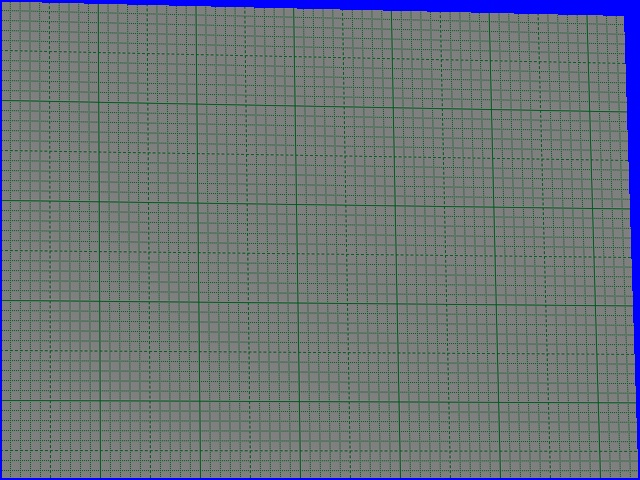images during test. Frame 1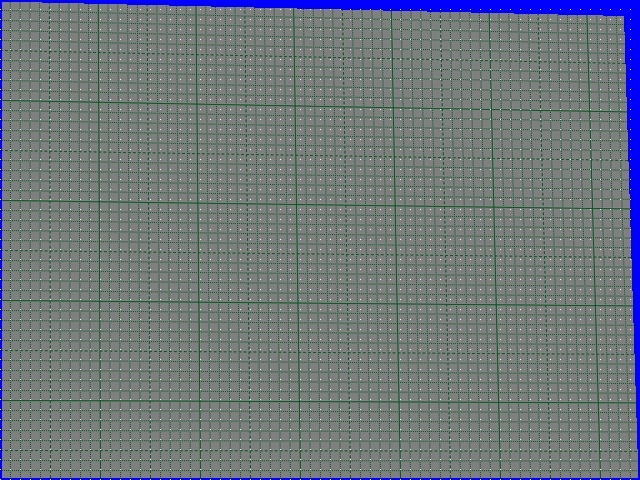frame 2. Good fit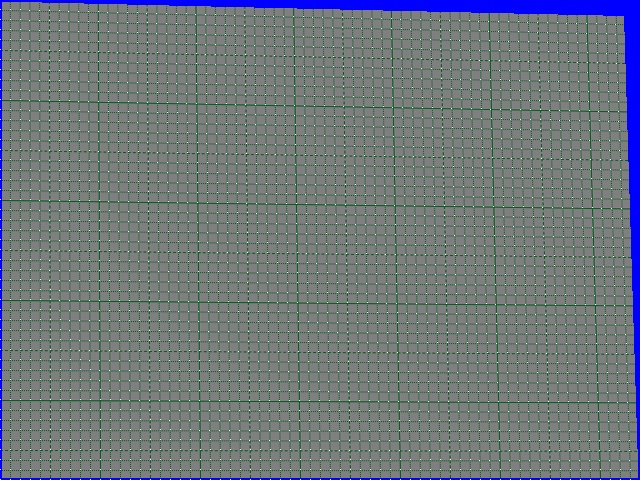Recovered Front view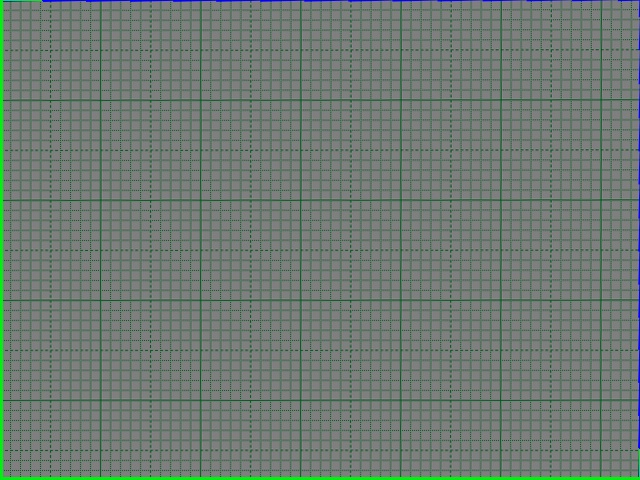for same input script line
Perspective(a= 0.0, b = -0.00010, x=0,y=300, color =\$fff)
outputs:-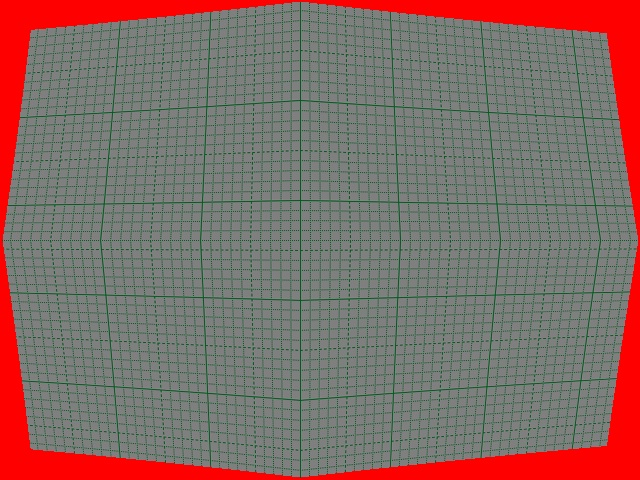script line
Perspective(a= 0.0005, x=300,y=240,z =0, color =\$ff)
outputs:-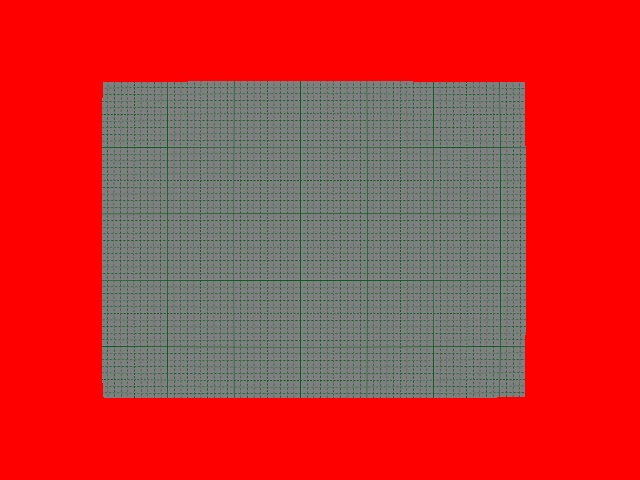script line
Perspective(a= -0.0005, x=300,y=240,z =0, color =\$ff)
outputs:-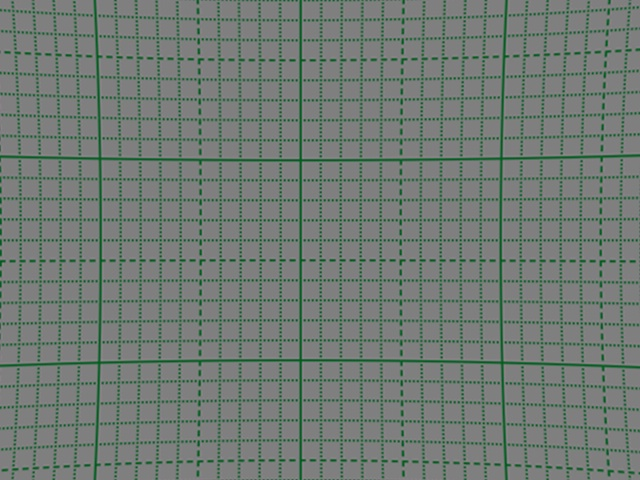>